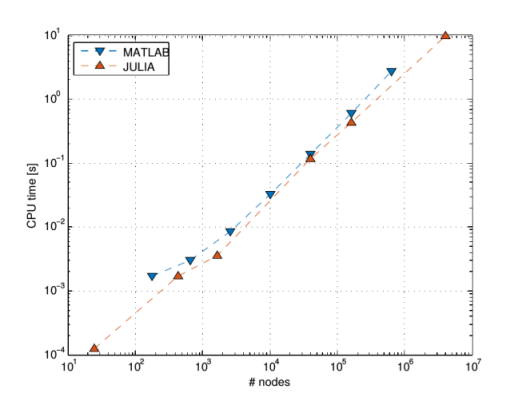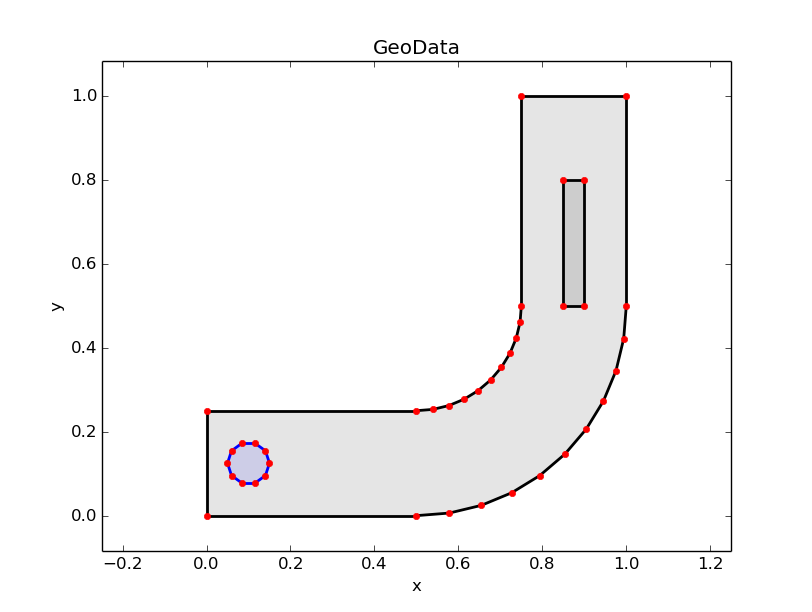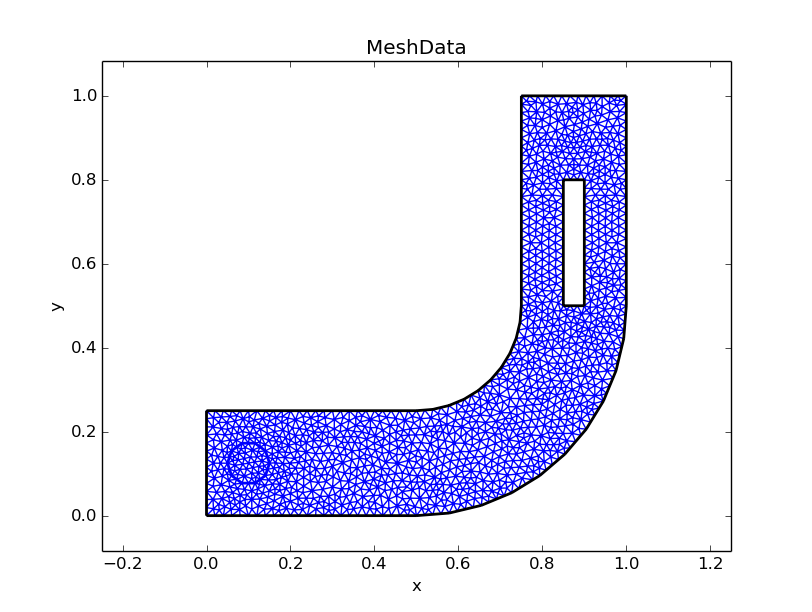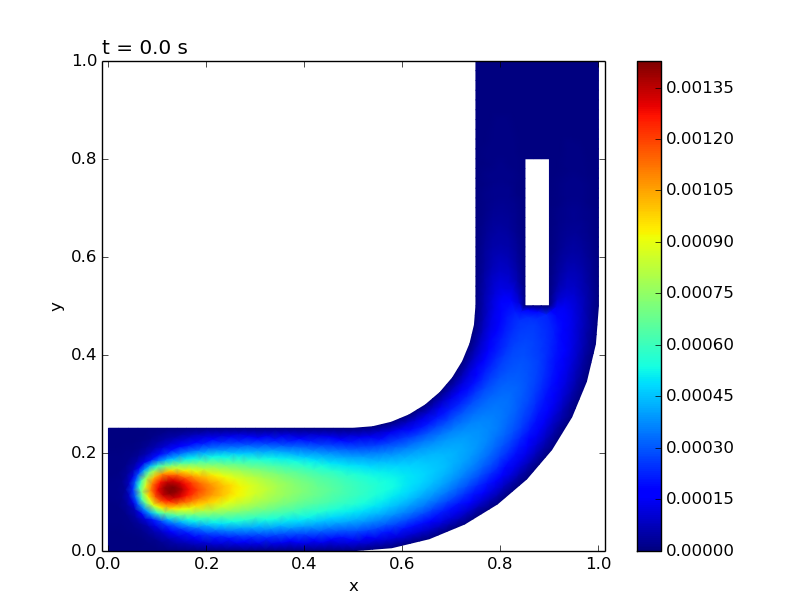3

0

6

3

# EllipticFEM.jl — a FEM Solver for Elliptic, Parabolic and Hyperbolic PDEs Written in JuliaThis package provides an implementation of a 2D finite element method for solving elliptic, parabolic or hyperbolic partial differential equations in Julia. In particular, one can solve

$(\partial_{t(t)} u) - \nabla (A*\nabla u) + b*\nabla u + c*u = f$  in $\Omega$,

$u = g\_D$ on $\Gamma\_D$,

$(A*\nabla u)*n = g\_N$ on $\Gamma\_N$,

$u = u$ on $\Gamma\_P$,


where $\Omega$ is the domain and $\Gamma_D$, $\Gamma_N$ and $\Gamma_P$ denote Dirichlet, Neumann and periodic boundary, respectively. The term $(\partial_{t(t)} u)$ can be either absent (elliptic equation), or one of $\partial_t u$ (parabolic equation) or $\partial_{tt}$ u (hyperbolic equation).

It is possible to prescribe

• Very general domains $\Omega$ with holes and subdomains.
• Arbitrary coefficients $A,b,c,f$ depending on space and time (defined on each subdomain independently).
• Arbitrary Dirichlet, Neumann and/or periodic boundary conditions depending on space and time (defined on each part of the boundary independently).

# Comparison to MATLAB

An important feature of this implementation in Julia is that the core matrix assembly is faster than in MATLAB (see Figure).You need:

# How to get started

The folder Run contains the subfolders Elliptic, Homogenization, Parabolic and Hyperbolic, each of which contains a runner file runElliptic.jl, runHomogenization.jl, runParabolic.jl or runHyperbolic.jl, respectively. Simply set your working directory to the respective path, start Julia and enter include("runElliptic.jl") in the Julia REPL.

The output images/videos are stored in the Saved images folder.

# Important data types

• type EquationData: Contains all the information about the problem the user wants to solve, i.e. the geometry of the domain including holes and subdomains, the coefficients A,b,c,f and the boundary conditions.
• type GeoData: Is an intermediate step between EquationData and MeshData. The boundaries of the domain, the holes and the subdomains are discretized and stored here. This type also handles the intersection points between intersecting subdomains. This type mimicks the data types used in gmsh, but adds some information to them.
• type MeshData: Contains all information about the triangular grid on the domain. The output of gmsh gets written into this data structure. Some additional geometric properties (e.g. the volume of each triangle) are computed and also stored here.
• type LSEData: Contains system matrix and load vector of the LSE occuring in the P1-FEM (i.e. piecewise linear Ansatz functions) for the aforementioned elliptic, parabolic or hyperbolic PDEs. The discrete solution(s) are stored here as well.

# An example (Elliptic equation)

The file runElliptic.jl essentially contains the following Julia code:

equationData = equationDataAssemble("./ellipticEquationDataLPipe.jl")

geoData = equationDataToGeoData(equationData)
geoDataPlot(geoData)

intendedMeshsize = 0.02
meshData = geoDataToMeshData(geoData,intendedMeshsize)
meshDataPlot(meshData)

deltaT = 0.0
lseData = lseDataAssemble(equationData,meshData,deltaT)

lseDataSolve(equationData,meshData,lseData)

lseDataPlot(meshData,lseData)

• The function equationDataAssemble(...) reads the equationData from the file ./ellipticEquationDataLPipe.jl. (You can edit this file to change the geometry of the domain or choose other coefficient functions $A,b,c,f,...$)
• The function equationDataToGeoData(...) discretizes the geometry defined in equationData and handles the intersection points between domain/holes/subdomains.
• The function geoDataPlot(...) plots the discretized geometry stored in geoData.
• The function geoDataToMeshData(...) runs gmsh on the discretized domain and stores the output in meshData.
• The function meshDataPlot(...) plots the triangular mesh generated by gmsh.
• The function lseDataAssemble(...) assembles the system matrix and the load vector of the LSE occuring in the FEM.
• The function lseDataSolve(...) solves the LSE defined in lseData and stores the solution(s) in lseData.
• The function lseDataPlot(...) plots the solution and eventually saves the resulting images/videos in the folder Saved images.03/02/2016

4 months ago

41 commits